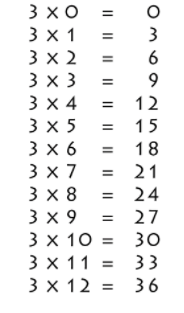# 3 Times Table- Learn Table Of 3 : Multiplication Table of 3

Safalta expert Published by: Yashaswi More Updated Thu, 26 May 2022 01:52 AM IST

## Highlights

Check out how to learn the multiplication table of 3 easily here at safalta.com

### Free Demo Classes

3 Times Table is the multiplication table of the prime number 3 with other numbers. Table of 3 can be calculated by repeated addition like 3 + 3 = 6 which is equivalent to 3 × 2. Learning table of 3 helps the child to gain a deeper understanding of multiplication which is helpful in the long run. Times tables prove to be an invaluable brain-training activity for young children. It is essential for students to memorize the 3 times table for quick calculations. Join Safalta School Online and prepare for Board Exams under the guidance of our expert faculty. Our online school aims to help students prepare for Board Exams  by ensuring that students have conceptual clarity in all the subjects and are able to score their maximum in the exams.

1.

Source: Safalta.com

Multiplication table of 3

2. Tips for 3 times table
3. Table of 3 from 11 to 20

Multiplication Table of 3

Learning a 3 times table is an essential skill for long division and multiplication. It helps in working out calculations in the head and makes problem-solving faster. Multiplication table of 3 helps in understanding the patterns of multiples. Go through the 3 times table chart that is given below for fast calculations.

### Multiplication table 3 chart## Tips for 3 Times Table

1. 3 doesn’t have any rules that make the multiplication table of 3 easy to memorize, but there is a pattern for every ten multiples of three: 3, 6, 9, 12, 15, 18, 21, 24, 27, 30.

2. The last digit of these multiples always repeat, which means that students can remember these digits to help them with the 3 times table.

### 3 × 20 = 60

Here are some example relating to the 3 times table-

1. ### Example 1: Using 3 times table, evaluate 3 times 4 minus 2?

Solution:

First, we will write 3 times 4 minus 2 mathematically.

Form table of 3, we have: 3 times 4 minus 2 = 3 × 4 - 2 = 12 - 2 = 10

Hence, 3 times 4 minus 2 is 10.

2. ### Example 2: Using the table of 3, Find the value of 2 plus 3 times 9 minus 6.

Solution:

First, we will write 2 plus 3 times 9 minus 6 mathematically.

Form 3 times table, we have: 2 plus 3 times 9 minus 6 = 2 + 3 × 9 - 6 = 2 + 27 - 6 = 23

Hence, 2 plus 3 times 9 minus 6 is 23.

3. ### Example 3: Find the value of 2 plus 3 times 9.

Solution:

First, we will write 2 plus 3 times 9 mathematically.

Using the table of 3, we have: 2 plus 3 times 9 = 2 + 3 × 9 = 2 + 27 = 29

Hence, 2 plus 3 times 9 is 29.

## Multiplication Tables

 2 Times Table 11 Times Table 3 Times Table 12 Times Table 4 Times Table 13 Times Table 5 Times Table 14 Times Table 6 Times Table 15 Times Table 7 Times Table 16 Times Table 8 Times Table 17 Times Table 9 Times Table 18 Times Table 10 Times Table 19 Times Table 20 Times Table

## What is the importance of learning Table 2 to 20?

For making mathematical section easier, memorising table 2 to 20 is important.

## How can I learn tables easily?

Daily recite the table as mentioned in the article twice and thrice.

## What is the value of 3 X 9?

The value of 3 X 9 is 27.

## What is the value of 3 X 7?

The value of 3 X 7 is 21.

## Start Learning & Earning

### Trending Courses##### Master Certification in Digital Marketing Programme (Batch-7)

Now at just ₹ 64999 ₹ 12500048% off##### Professional Certification Programme in Digital Marketing!

Now at just ₹ 49999 ₹ 9999950% off##### Advanced Certification in Digital Marketing Online Programme (Batch-18)

Now at just ₹ 24999 ₹ 3599931% off##### Advanced Certification in Digital Marketing CLASSROOM PROGRAMME

Now at just ₹ 44999 ₹ 9999955% off##### Basic Digital Marketing Course (Batch-23): 50 Hours Live+ Recorded Classes!

Now at just ₹ 1499 ₹ 999985% offNow at just ₹ 599 ₹ 159963% off##### Advance Graphic Designing Course (Batch-7) : 150 Hours of Learning

Now at just ₹ 12999 ₹ 2999957% off